# IB DP Physics: SL复习笔记4.3.5 Malus’s Law

### Malus's Law

#### Intensity of Polarised Light

• The intensity of unpolarised light is reduced as a result of polarisation
• If unpolarised light of intensity I0 passes through a polariser, the intensity of the transmitted polarised light falls by a half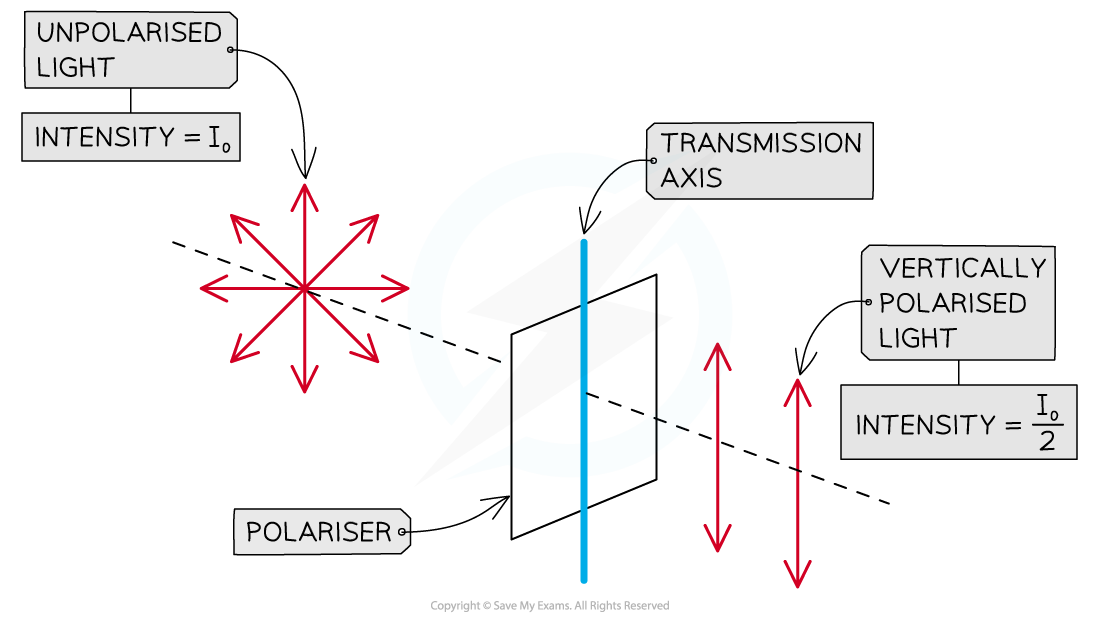The intensity of polarised light transmitted by a polariser is half the intensity of the unpolarised light incident on it

#### Intensity of Analysed Light

• The first filter that the unpolarised light goes through is the polariser
• A second polarising filter placed after the first one is known as an analyser
• If the analyser has the same orientation as the polariser, the light transmitted by the analyser has the same intensity as the light incident on it
• If they have a different orientation, we must use Malus's Law to determine the intensity of the transmitted light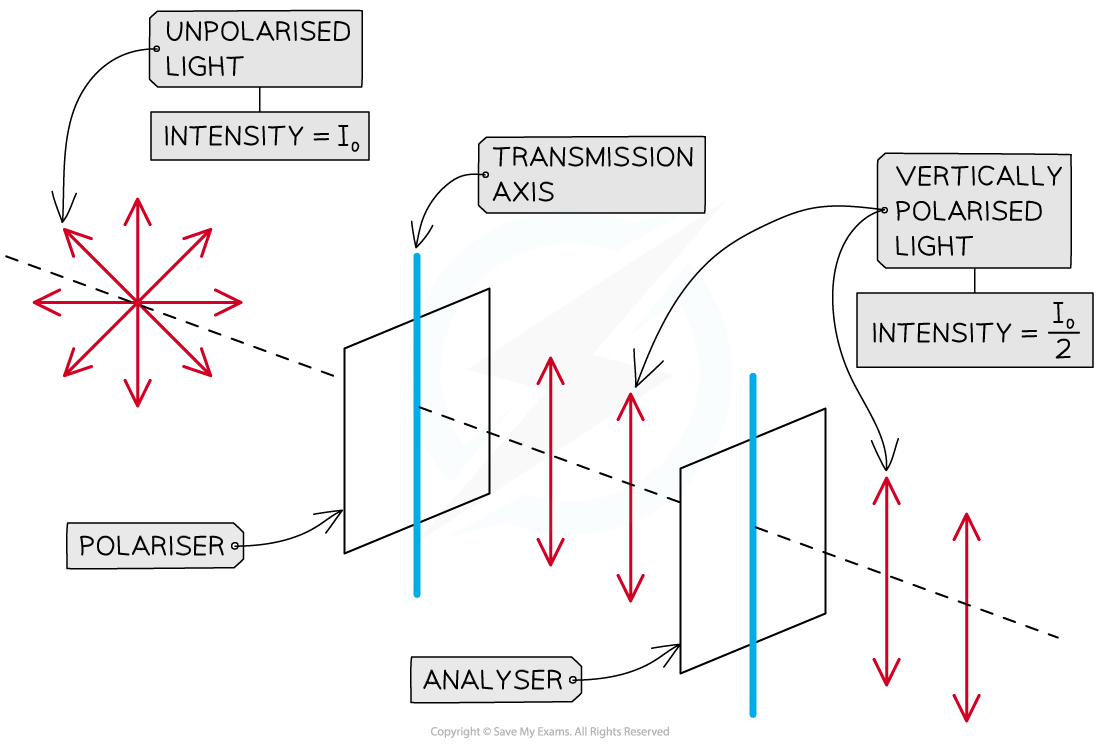When the polariser and the analyser have the same orientation (i.e. parallel transmission axes), the intensity of analysed light is the same as the intensity of polarised light

• Malus's Law states that if the analyser is rotated by an angle θ with respect to the polariser, the intensity of the light transmitted by the analyser is• If the polariser and the analyser have the same orientation, light transmitted by the analyser has the same intensity as light that is incident upon it, since cos(0) = 1
• If vertically polarised light with intensity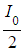is incident on an analyser with a vertical transmission axis, all of the light will be transmitted through the analyser
• The intensity will not decrease between the polariser and the analyser
• If the analyser is rotated by 90° with respect to the polariser (θ = 90°), the intensity of the light transmitted by the analyser will be zero, since cos(90°) = 0
• If vertically polarised light is incident on an analyser with a horizontal transmission axis, none of the light will be transmitted through the analyser
• In this instance, all the light will be absorbed

Table of transmitted intensity when vertically polarised light is incident upon an analyser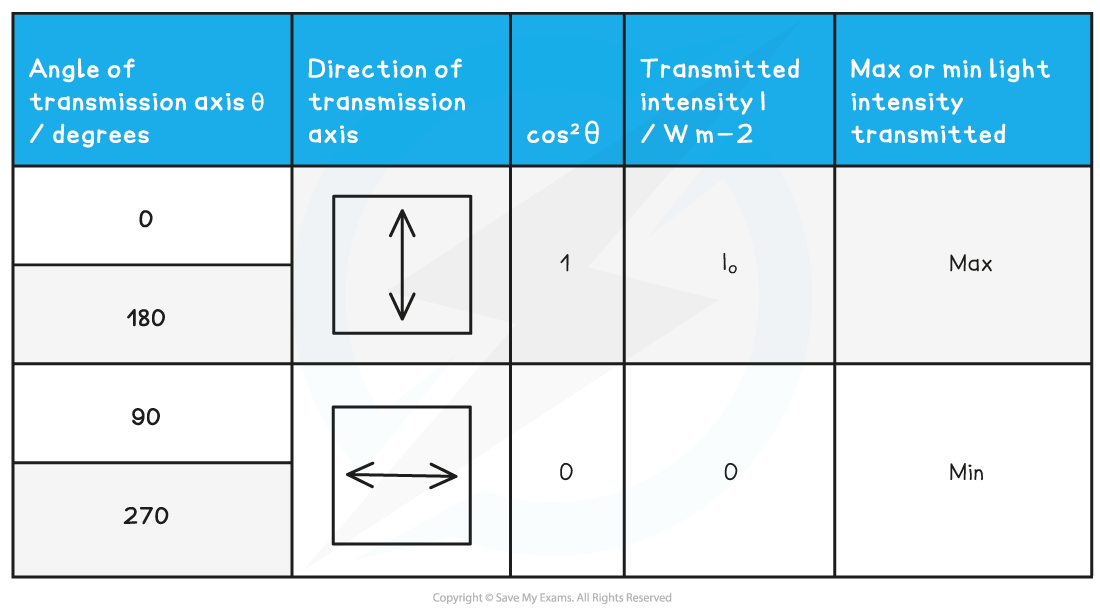• If the analyser has any other orientation with respect to the polarised light incident upon it, then Malus's Law is used to determine the intensity of the analysed light
•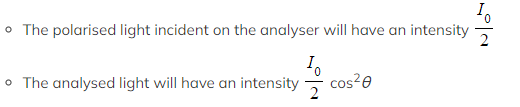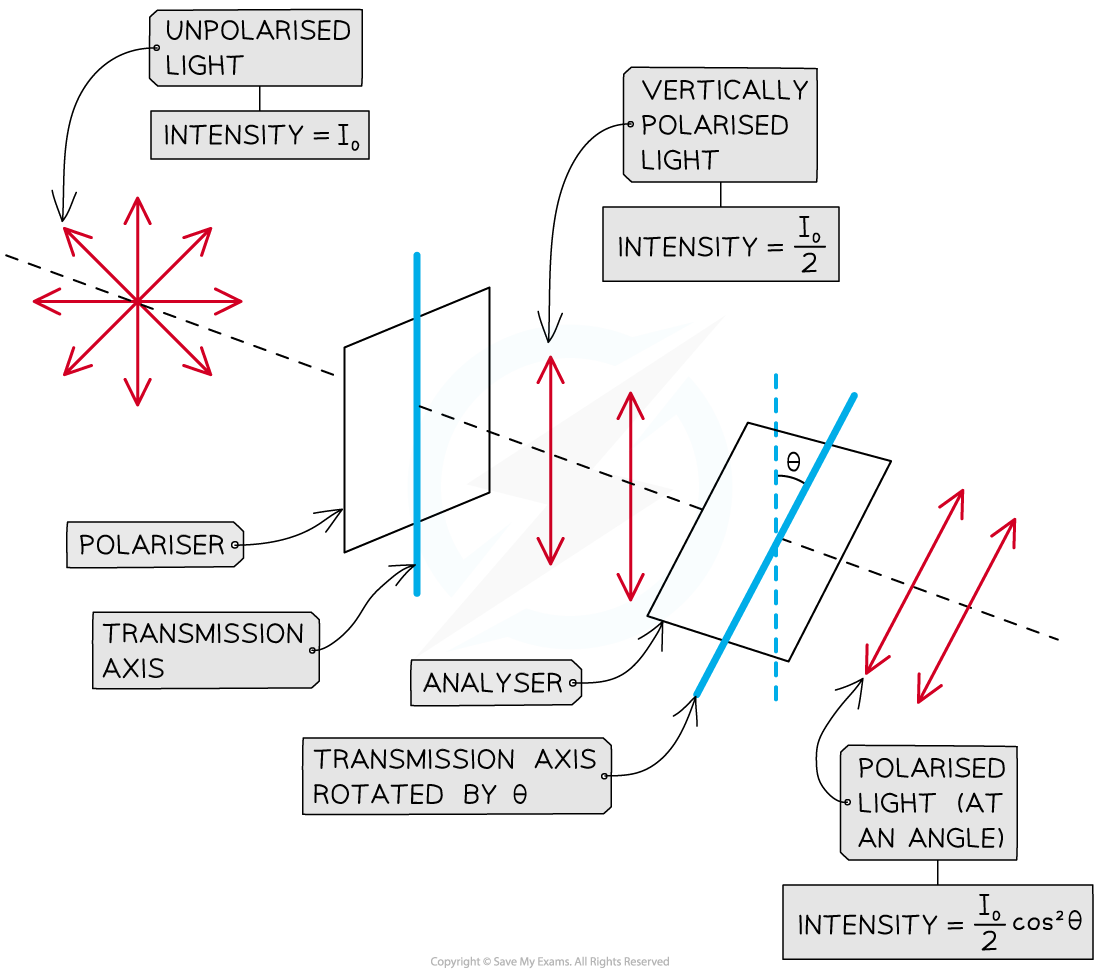When the analyser is rotated with respect to the polariser by an angle, the intensity of analysed light variates with cos2θ

• The resulting graph of the light intensity with angle, as the analyser is rotated through 360°, looks as follows: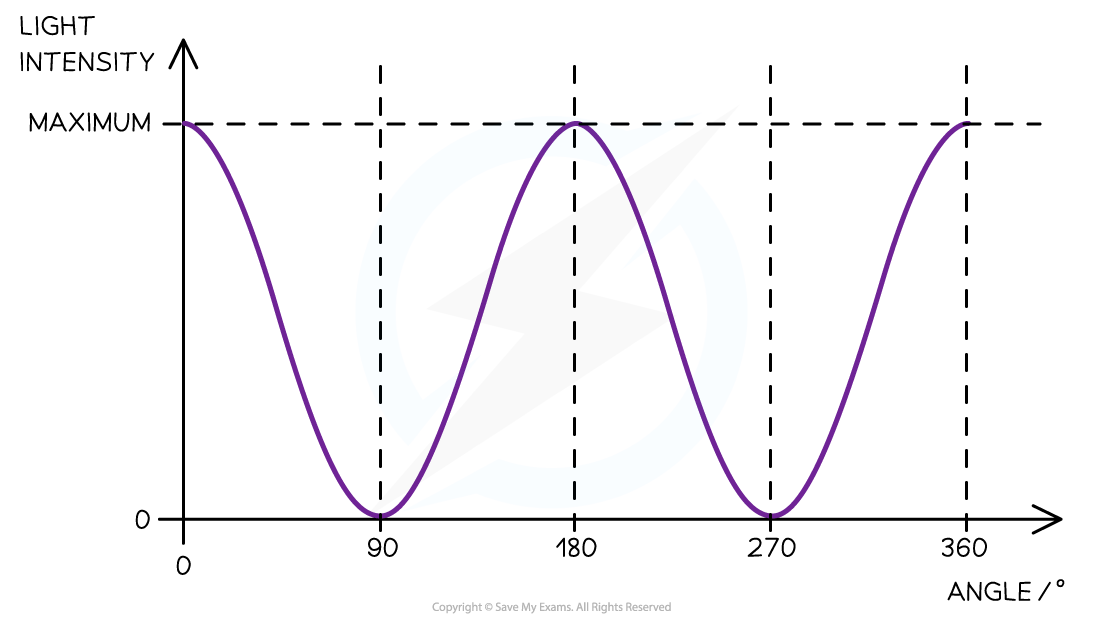Graph showing how the intensity of the analysed light beam varies with the angle between the transmission axes of the polariser and analyser

• The maximum light intensity I of the graph is still half of the intensity from the unpolarised light (I0)• The two extremes of maximum light intensity and minimum light intensity depend on the orientations of just one of the polarisers
• If an unpolarised light source is placed in front of two identical polarising filters, A and B, with their transmission axes parallel:
• Filter A will polarise the light in a certain axis
• All of the polarised light will pass through filter B unaffected
• In this case, the maximum intensity of light is transmitted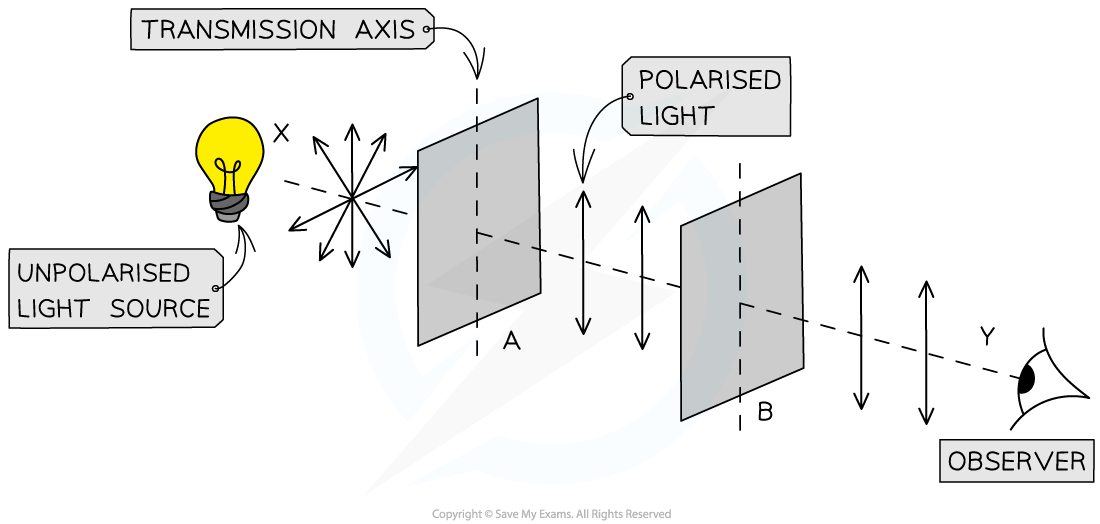When both polarisers have the same transmission axis, the intensity of the transmitted light is at its maximum

• As the polarising filter B is rotated anticlockwise, the intensity of the light observed changes periodically depending on the angle B is rotated through
• When A and B have their transmission axes perpendicular to each other:
• Filter A will polarise the light in a certain axis
• This time none of the polarised light will pass through filter B
• In this case, the minimum intensity of light is transmitted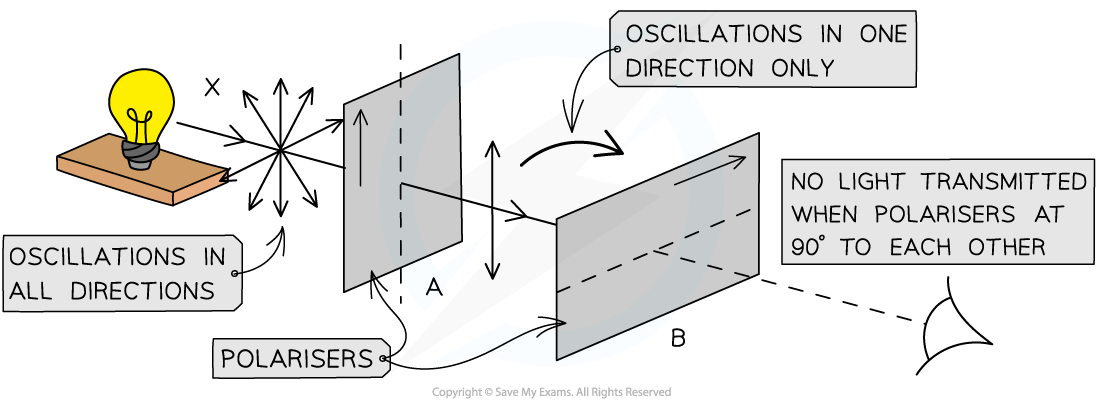When one of the polarisers is rotated through 90°, the intensity of the transmitted light drops to zero

#### Worked Example

Unpolarised light of intensity I0 is incident on a polariser. The transmitted polarised light is then incident on an analyser. The transmission axis of the analyser makes an angle of 45° with the transmission axis of the polariser.

Determine the intensity of light transmitted by the analyser.

Step 1: Write down the known quantities

• Intensity of unpolarised light = I0
• Angle of rotation of analyser with respect to polariser,  θ = 45°

Step 2: Write down Malus's law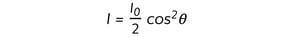Step 3: Substitute the value of the angle θ = 45°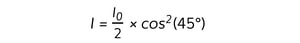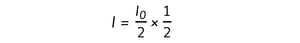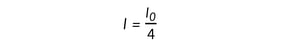• The intensity of light transmitted by the analyser is a quarter the intensity of unpolarised light
• (and one half the intensity of light transmitted by the polariser)

#### Exam Tip

Remember that the unpolarised light coming through will always halve in intensity when it becomes polarised through an polariser. Only then should you use Malus' law to find the intensity of the light after it has passed through the analyser. Therefore, the I and I0 in Malus' law are the intensities of light that are already polarised.